Himanshi Singh — Updated On April 6th, 2021

## Introduction

In this article, we’ll see what is Random Forest algorithm and also what are its hyper-parameters? Random forest is one of the most commonly used algorithms that work on the concept of bagging.

Note: If you are more interested in learning concepts in an Audio-Visual format, We have this entire article explained in the video below. If not, you may continue reading.

So let’s understand how the algorithm works. From a given data set, multiple bootstrap samples are created and the number of bootstrap samples would depend on the number of models we want to train. Suppose I want to build 10 models here then I’ll create 10 bootstrap samples-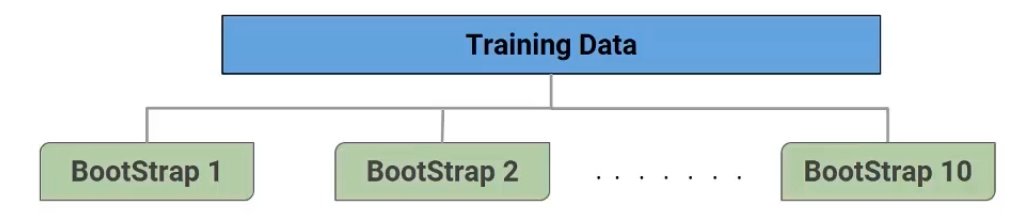Now on each of these bootstrap samples, we built a decision tree model. So here, as you can see, if we have 10 decision tree models, each built on a different subset of data-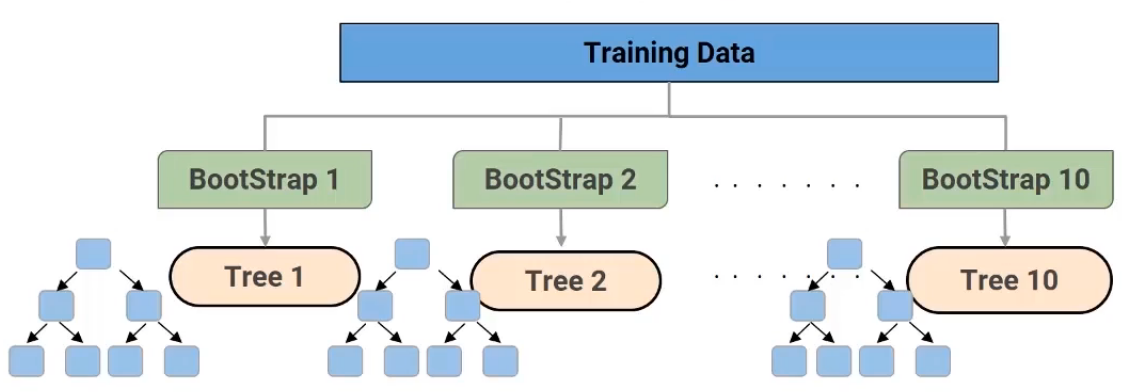Now each of these individual decision trees would generate a prediction, which is then finally combined to get the final output or the final prediction-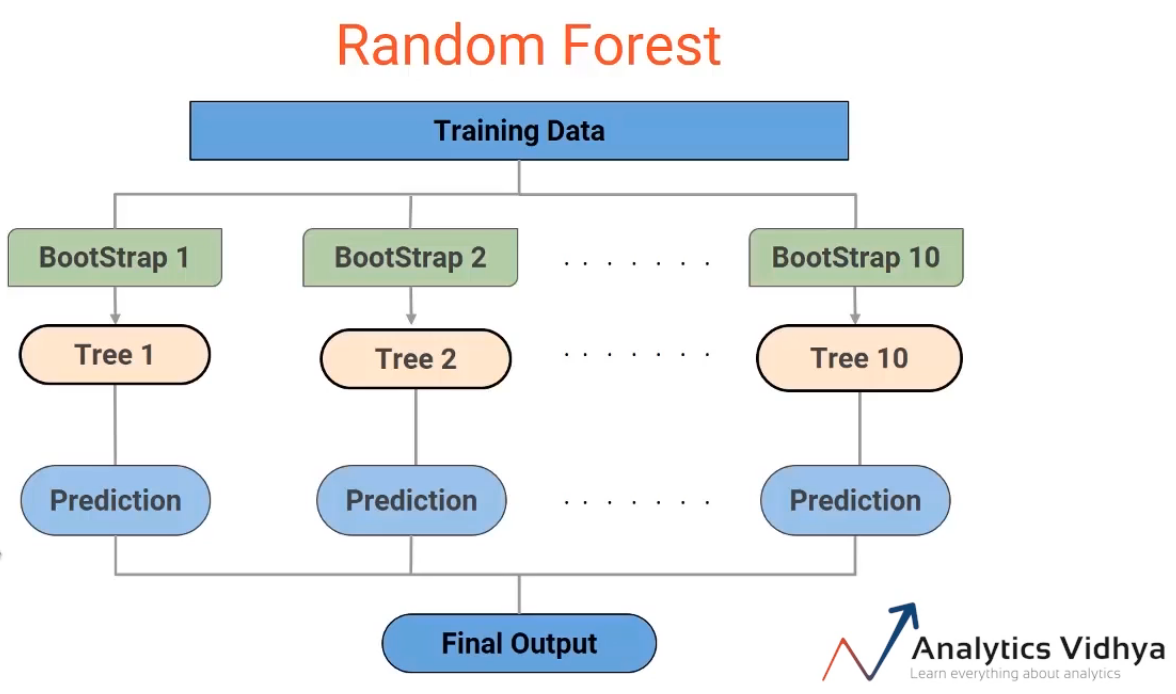So effectively we’re combining multiple trees to get the final output. And hence it’s called the forest. But why is it called Random Forest? You might say it’s because we use the random bootstrap samples. Well, that’s partially correct, along with a random sampling of data points or rows, the random forest also performs random sampling of features.

Note that the random sampling of rows is done at the tree level. So every tree will get a different subset of data points. Feature sampling is done on the node level or on the split level and not on the tree level.

So let’s dive deeper into this concept of feature sampling for the random forest. Let’s take an example. Suppose we have nine features in the data set from V1 to V9-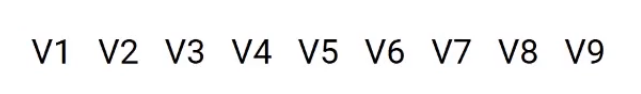Now for a simple decision tree model, we use all of these features to build the tree. So all the nine features are used at the first node to decide the best split-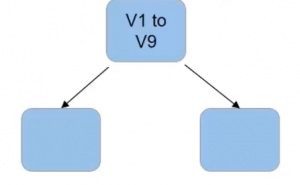And then again, all the nine features are used at the two leaf nodes to decide the best split-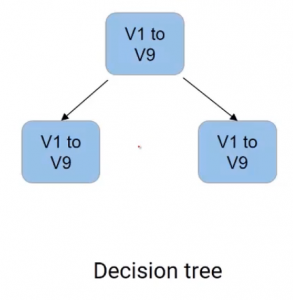but for a tree built-in random forest, a subset of features is selected at every node. So let’s say we have the first subsidy as follows V1 V3, V4, and V9-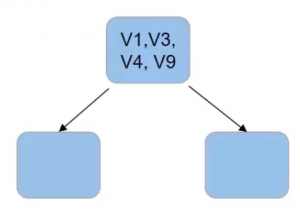Then these features will be used at the root node to decide the best split. And for the next node, we’ll create another subset of features, which is used for this further split-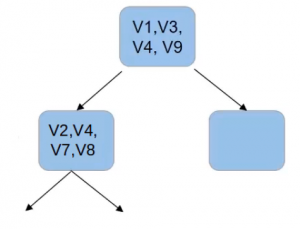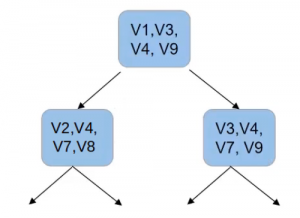And similarly, for the other node here, we’ll create a new subset of features, which will be used to make the split. And this process goes on for every node in every tree.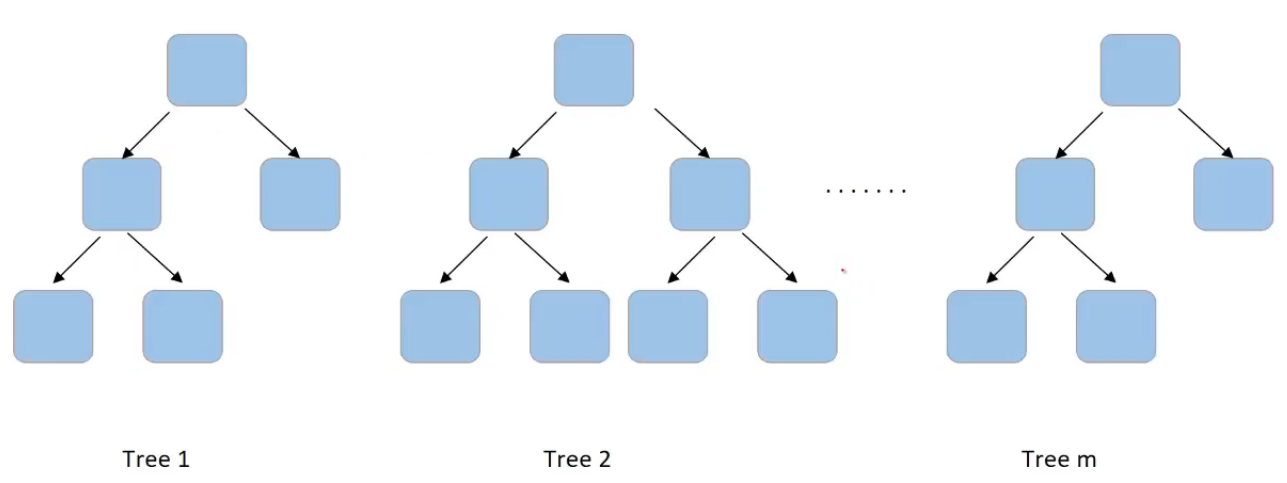So if we are building m trees, for each node in each tree, you select a subset of features and then choose the best split. Let’s have a quick recap of what we covered in random forest-

• So first we create multiple bootstrap samples and the number of samples would depend on the number of models we want to build.
• Then on each of these bootstrap samples, we train a decision tree model during the building of these decision trees. We perform feature sampling at every split.
• And finally, we aggregate all the decision trees to get the final output.

## Hyper-parameters of Random Forest

First, understand what the term hyper-parameters means? We have seen that there are multiple factors that can be used to define the random forest model. For instance, the maximum number of features used to split a node or the number of trees in the forest. We can manually set and tune these values. So I can set the number of trees to be 20 or 200 or 500. We can manually change and update these values. And these are called the hyper-parameters of random forest.

### 1. n_estimators: Number of treesLet us see what are hyperparameters that we can tune in the random forest model. As we have already discussed a random forest has multiple trees and we can set the number of trees we need in the random forest. This is done using a hyperparameter “n_estimators

### 2. max_features

There are multiple other hyper-parameters that we can set at a tree level. So consider this one tree in the forest-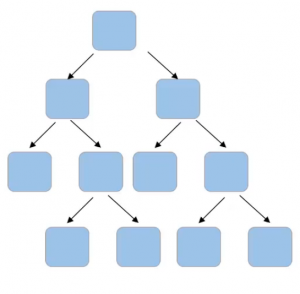Can you think of hyper-parameters for this tree? Firstly, if we can set the number of features to take into account at each node for splitting, for example-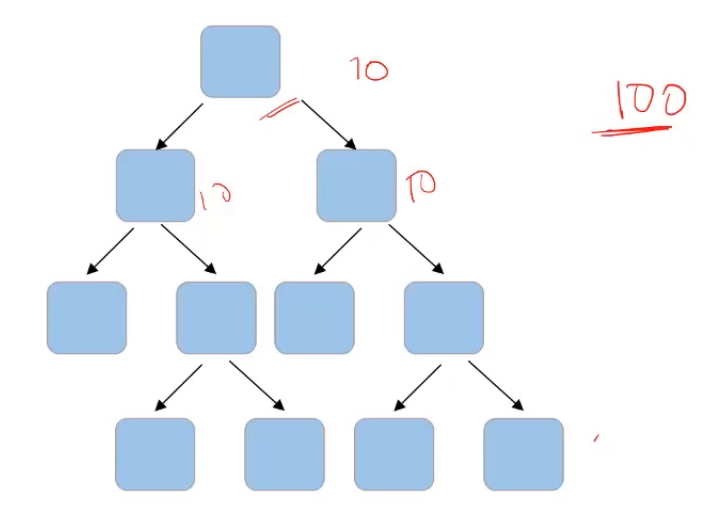If the total number of features in my data set is a hundred and I decide that I’ll randomly select the square root of these total number of features. So which comes out to be 10. So I’ll randomly select 10 features for this node and the best feature will be used to make the split. Then again, I’ll randomly select 10 features for another node. And similarly, for all the nodes, instead of taking a square root, I can also consider taking the log of the total number of features in the dataset. So “max_features” is one of the parameters that we can tune to randomly select the number of features at each node.

### 3. max_depth

Another hyperparameter could be the depth of the tree. For example, in this given tree here,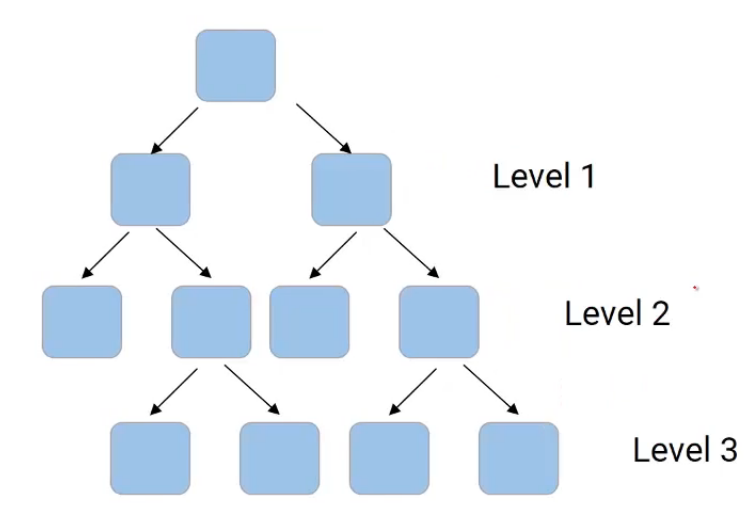we have level one, we have level two, and a level three. So the depth of the tree, in this case, is three. We can decide the depth to which a tree can grow using “max_depth“, and this can be considered as one of the stopping criteria that restrict the growth of the tree.

### 4. min_samples_split

Another value that we can control is the minimum number of samples required to split a node and the minimum number of samples at the leaf node. So for instance, if I have a thousand data points and after the first split, I have 70o in one node and 300 in the other node. And here are the values of the number of data points in each of the node after splitting-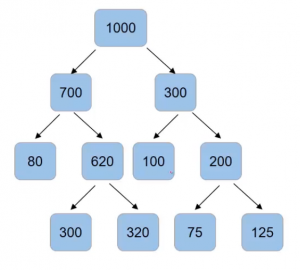Now we can set the criteria that a normal split only when the number of samples in the node is more than 100 using “min_samples_split“. So node with 80 samples will not have for the split, same as the case with a node with 100 and 75 samples since our condition is that the number of samples should be more than 100.

### 5. min_samples_leaf

Another condition that we can set is the number of samples in the leaf node using “min_samples_leaf“. So if I save that the split can happen only when after the split, the number of samples in the leaf node comes out to be more than 85. Then, in that case, this will be an invalid split since, after the split, the number of samples is only 80-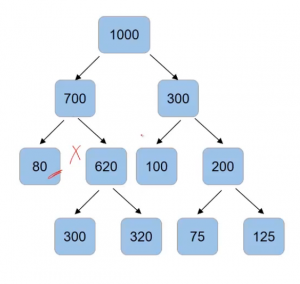The split on 300 and 620 are valid but the split on 200 will not take place-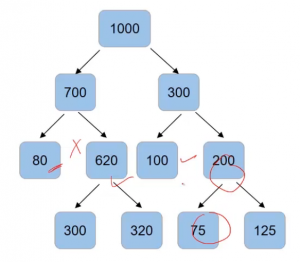### 6. criteria

Another important hyper-parameter is “criteria“. While deciding a split in decision trees, we have several criteria such as Gini impurity, information gain, chi-square, etc. Similarly, we can have some other criteria like entropy. So to set, which criteria to use will be done using the hyperparameter “criteria”.

So these were the most important hyper-parameters of random forest.

## End Notes

In this article, we learned about the random forest algorithm and some of its important parameters which you should know before diving further into the details of this algorithm.

If you are looking to kick start your Data Science Journey and want every topic under one roof, your search stops here. Check out Analytics Vidhya’s Certified AI & ML BlackBelt Plus Program

If you have any queries, let me know in the comment section!